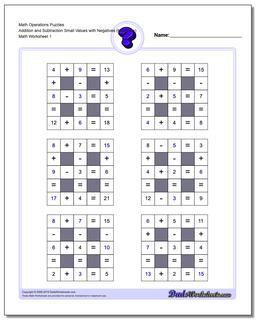# Math Worksheets: Number Grid Puzzles: Number Grid Puzzles: Math Operations Puzzles Addition and Subtraction Small Values with Negatives (Moderate)## Math Operations Puzzles Addition and Subtraction Small Values with Negatives (Moderate)

PropertyValue
DescriptionMath Operations Puzzles Addition and Subtraction Small Values with Negatives (Moderate): Math puzzle worksheets that require students to fill in missing numbers with smaller numbers.
Resource TypeWorksheet